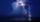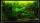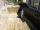Pool

The swimming pool is 10 m wide and 8 m long and 153 cm deep. How many hectoliters of water is in it, if the water is 30 cm below its upper edge?

Result

V =  984 hl

Solution:Leave us a comment of example and its solution (i.e. if it is still somewhat unclear...):Math student
IT'S 9 CM NOT 10 CM. SO IT SHOULD BE 4004.Dr Math
corrected, thank youTo solve this example are needed these knowledge from mathematics:

Do you want to convert length units? Do you know the volume and unit volume, and want to convert volume units?

Next similar examples:

1. Swimming pool 4The pool shaped cuboid measuring 12.5 m × 640 cm at the bottom is 960hl water. To what height in meters reaches the water level?
2. Water poolWhat water level is in the pool shaped cuboid with bottom dimensions of 25 m and 10 meters, when is 3750hl water in the pool.
3. Water tank 2Water tank cuboid is 12 meters long and 6.5 meters wide and 1.2 meters high. How many hectoliters are in the tank when it is filled to 81%?
4. Plan scaleAt what scale is drawn plan of the building, where one side of the building is 45 meters long is on the plan expressed by a straight line 12 mm long.
5. Water lakeThe length of the lake water is 8 meters width 7 meters and depth 120 centimeters. How many liters of water can fit into the water lake?
6. Reservoir 3How many cubic feet of water is stored in the reservoir that has a length of 200 feet, a width of 100 feet, an overflow depth of 32 feet, and a current water level of 24 feet?
7. WaterOn the lawn which has area 914 m2 rained 3 mm of water. How many liters of water rained?
8. RainIt rains at night. On 1 m2 of lake will drop 60 liters of water. How many cm will the lake level rise?
9. Feet to milesA student runs 2640 feet. If the student runs an additional 7920 feet, how many total miles does the student run?
10. Concrete boxThe concrete box with walls thick 5 cm has the following external dimensions: length 1.4 m, width 38 cm and height 42 cm. How many liters of soil can fit if I fill it to the brim?
11. Aquarium volumeThe aquarium has a cuboid shape and dimensions a = 0.3 m, b = 0.85 m, c =? , V = ?. What volume has a body, if after dipping into the aquarium water level rises by 28 mm?
12. Water tankWater tank shape cuboid has a width of 3.1 m and length twice larger. How high will reach water if water flow into 13 liters of water per second during 16 minutes?
13. Water levelHow high reaches the water in the cylindrical barell with a diameter of 12 cm if there is a liter of water? Express in cm with an accuracy of 1 decimal place.
14. An oilAn oil drum is cut in half. One half is used as a water trough. Use the dimensions; length 82cm, width 56cm to estimate the capacity of the water trough in liters.
15. Water levelThe glass container has a cuboid shape with dimensions of the bottom 24 cm and 12 cm. The height of water is 26 cm. Calculate the volume of the body, which are sunk into the water if the water level rise by 3 cm.
16. DiggingA pit is dug in the shape of a cuboid with dimensions 10mX8mX3m. The earth taken out is spread evenly on a rectangular plot of land with dimensions 40m X 30m. What is the increase in the level of the plot ?
17. ExcavationExcavation for the base of the cottage 4.5 m x 3.24 m x 60 cm. The excavated soil will increase its volume by one-quarter. Calculate the volume of excavated soil.